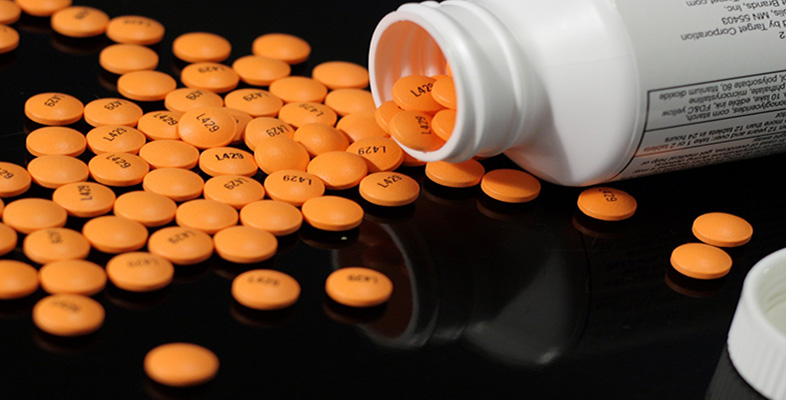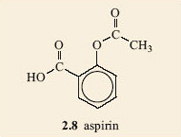Pain and Aspirin

Start this free course now. Just create an account and sign in. Enrol and complete the course for a free statement of participation or digital badge if available.

Free course

# 4.3 Aspirin

## Question 1

Compare the structure of aspirin, 2.8, with that of salicylic acid, 2.7. What similarities and differences can you see?The structures look quite similar. They both have a benzene ring carrying two groups, on adjacent carbon atoms. In both of them one of the groups is a carboxylic acid group. But, salicylic acid carries a phenol group whilst aspirin does not.

## Question 2

Can you identify the group that is carried by aspirin in the corresponding place to the phenol group in the molecule of salicylic acid? Have a look at options in Table 1.

You should have concluded that this is an ester group. If you did not identify this group correctly, try making a model. Remember there is free rotation about the single bonds and this should enable you to make it look like the ester group in Table 1.

You are going to study an important reaction between functional groups on molecules in this section. If you are new to chemistry you may not have seen chemical equations before, so before moving on work through Box 1 which provides you with a brief introduction to this topic.

## Box 1 An introduction to chemical equations

When chemists want to refer to a chemical reaction in which bonds are broken and new bonds are formed to produce new molecules (the products) from other molecules (the reactants), they often do so by means of a chemical equation. The reactants are shown on the left and the products are on the right,

reactants = products

As atoms cannot be created or destroyed in chemical reactions, the total number of atoms of each element involved must be the same on each side of the equation, if the equation is to ‘balance’ correctly. The two sides are then linked by an equals (=) sign and the reaction is referred to as a ‘balanced reaction’.

For example, methane – the first molecule you made a model of and a fossil fuel gas – burns in the oxygen of the air to form carbon dioxide (CO2) and water (H2O). The reaction can be represented by the equation:

CH4 + 2O2 = CO2 + 2H2O

Count the atoms of each element on the left of the reaction and compare with the numbers on the right. They should be the same! Remember 2O2 means 2 × 2 = 4 atoms of oxygen (O).

Note the need for two molecules of oxygen and two molecules of water to balance the equation. The information that this equation contains is that one molecule of methane reacts with two molecules of oxygen to produce one molecule of carbon dioxide and two molecules of water.

Sometimes the numbers of the molecules are unimportant and we just want to focus on the formulae of the reactants and products, not how much of each is involved. Chemists often show this type of relationship with a reaction having an arrow (→) instead of an equals sign. The following reaction is in this style:

CH4 + O2H2O

SK185_1